Appendix B: Linearization and expansion by the Fourier series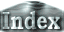We linearize (1) and (2) assuming small amplitudes for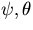. Further, we expandby a series of functions satisfying the boundary conditions such that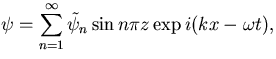(5)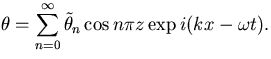(6)

Operating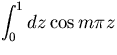on and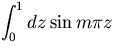on the linearized equations of (2) and (1), respectively, we obtain equations of an eigenvalue problem of infinite dimension for eigenvalue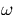and eigenvector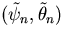.(7)

where <..|..> denotes the inner product of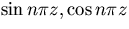.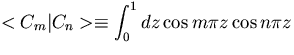,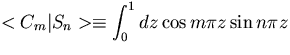,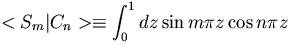,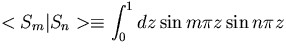.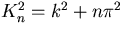is the square of the total wave number for the vertical wave number n.

In order to solve (7) analytically, we investigate the truncated system of (7) up to the vertical wave number 1. We believe that the qualitative characteristics of the solutions are conserved in this truncation, although the eigenvalues and structures of eigenvectors may be quantitatively different from those of exact solutions. (7) becomes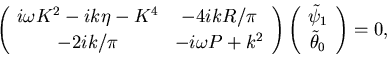(8)

where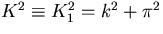is a square of the total wave number for the vertical wave number 1. By evaluating the determinant of the coefficient matrix, we obtain the following dispersion relation of the system: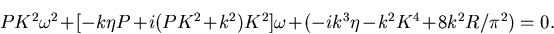(9)

In order to investigate the critical states, we assume thatis a real number. From the imaginary part of (9) we have the expression of frequency for neutral modes,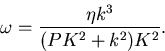(10)

By substituting (10) into the real part of (9), we obtain the equation of neutral curves.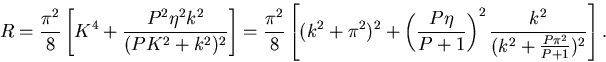(11)

 Appendix B: Linearization and expansion by the Fourier series